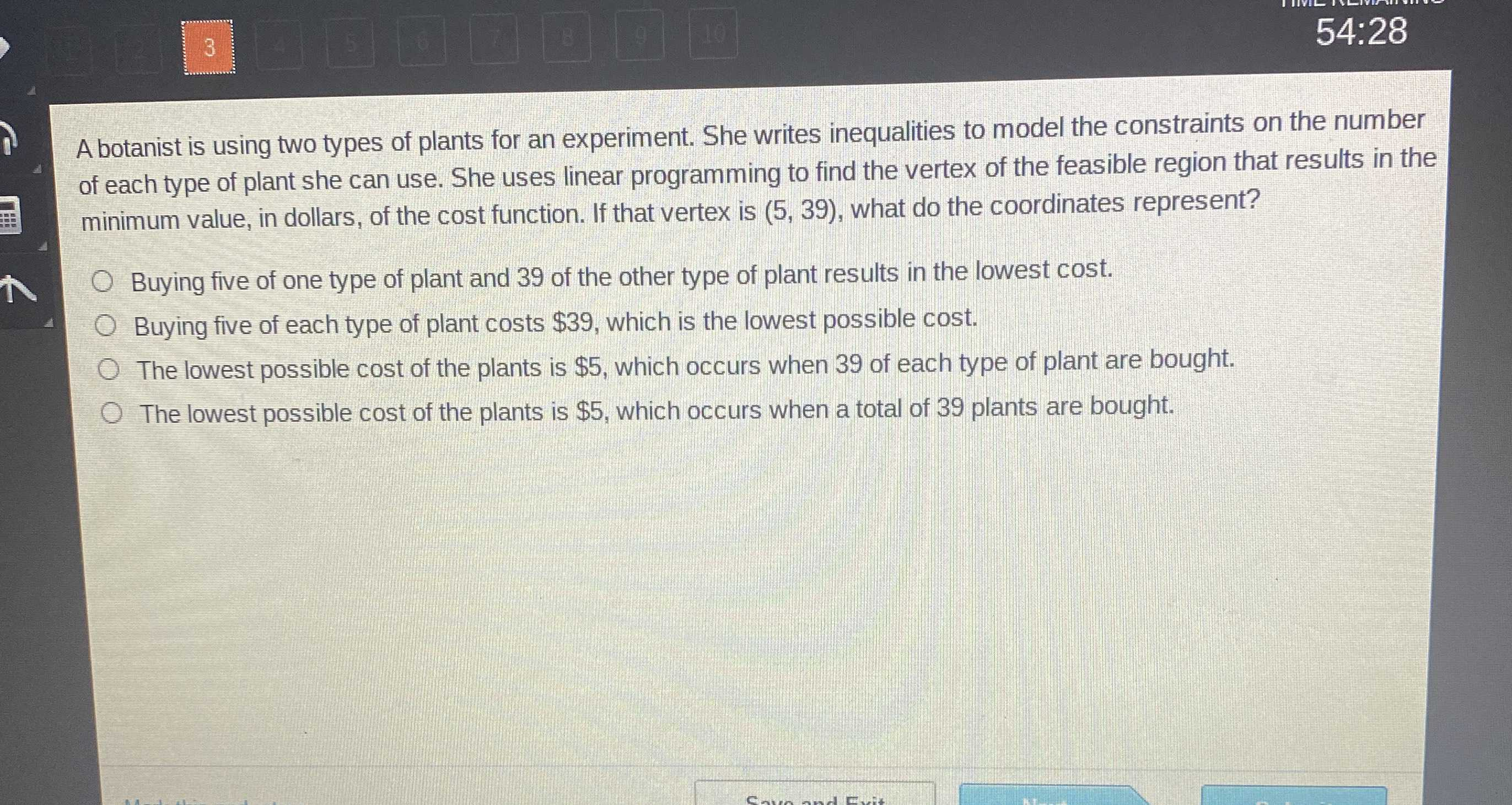### ¿Todavía tienes preguntas de matemáticas?

Pregunte a nuestros tutores expertos
Algebra
PreguntaA botanist is using two types of plants for an experiment. She writes inequalities to model the constraints on the number of each type of plant she can use. She uses linear programming to find the vertex of the feasible region that results in the minimum value, in dollars, of the cost function. If that vertex is (5, $$39$$ ), what do the coordinates represent?

Buying five of one type of plant and $$39$$ of the other type of plant results in the lowest cost.

Buying five of each type of plant costs $$\ 39 ,$$ which is the lowest possible cost.

The lowest possible cost of the plants is $$\ 5 ,$$ which occurs when $$39$$ of each type of plant are bought. The lowest possible cost of the plants is $$\ 5 ,$$ which occurs when a total of $$39$$ plants are bought.

Buying five of each type of plant costs $$\ 39 ,$$ which is the lowest possible cost.